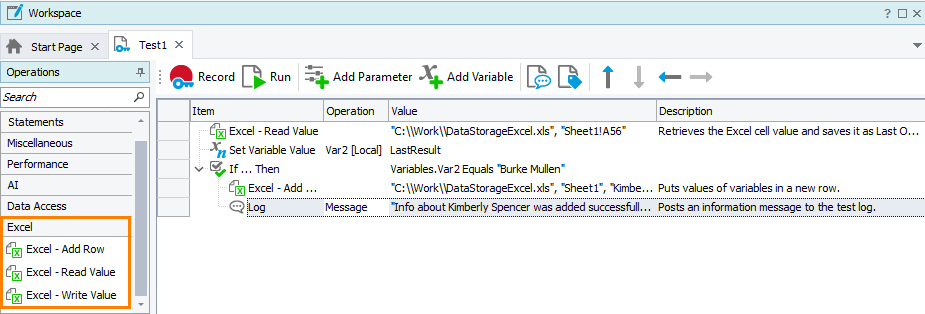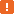# Working with Microsoft Excel FilesWhen testing, you may need to use data from external storages. Microsoft Excel files are among the most popular data storages. TestComplete allows you to retrieve data from Excel files and use them in your tests. Also, you can add data to existing Excel files or create new ones.

### In Keyword Tests

Note: To work with Excel files in your tests, you do not need to have Microsoft Office Excel installed on your computer.

Use special operations grouped into the Excel section to read data from Excel cells and write data to them.When you write data via these operations, you do not need to add additional operations to save changed data. Excel operations will do it automatically.

### In Script Tests

If you work with Excel files in script tests, use the `Excel` object. The following script illustrates how to read data from Excel cells and write it into a new row:

JavaScript, JScript

function ExcelExample()
{
// Get the sheet of the Excel file
var excelFile = Excel.Open("C:\\temp\\DataStorageExcel.xlsx");
var excelSheet = excelFile.SheetByTitle("Sheet1");

// Read data from the Excel file
var valueA = excelSheet.Cell("A", 3).Value;
var valueB = excelSheet.Cell(2, 3).Value;
var valueC = excelSheet.CellByName("C3").Value;

// Write the obtained data into a new row of the file
var rowIndex = excelSheet.RowCount + 1;
excelSheet.Cell("A", rowIndex).Value = valueA;
excelSheet.Cell(2, rowIndex).Value = valueB;
excelSheet.Cell("C", rowIndex).Value = valueC;

// Save the file to apply the changes
excelFile.Save();

// Save the file with another name
// excelFile.SaveAs("C:\\temp\\DataStorageExcel_new.xlsx");
}

Python

``````def ExcelExample():

# Get the sheet of the Excel file
excelFile = Excel.Open("C:\\temp\\DataStorageExcel.xlsx")
excelSheet = excelFile.SheetByTitle["Sheet1"]

# Read data from the Excel file
valueA = excelSheet.Cell["A", 3].Value
valueB = excelSheet.Cell[2, 3].Value
valueC = excelSheet.CellByName["C3"].Value

# Write the obtained data into a new row of the file
rowIndex = excelSheet.RowCount + 1
excelSheet.Cell["A", rowIndex].Value = valueA
excelSheet.Cell[2, rowIndex].Value = valueB
excelSheet.Cell("C", rowIndex).Value = valueC

# Save the file to apply the changes
excelFile.Save()

# Save the file with another name
# excelFile.SaveAs("C:\\temp\\DataStorageExcel_new.xlsx")``````

VBScript

Sub ExcelExample
Dim excelFile, excelSheet, valueA, valueB, valueC, rowIndex

' Get the sheet of the Excel file
Set excelFile = Excel.Open("C:\temp\DataStorageExcel.xlsx")
Set excelSheet = excelFile.SheetByTitle("Sheet1")

' Read data from the Excel file
valueA = excelSheet.Cell("A", 3).Value
valueB = excelSheet.Cell(2, 3).Value
valueС = excelSheet.CellByName("C3").Value

' Write the obtained data into a new row of the file
rowIndex = excelSheet.RowCount + 1
excelSheet.Cell("A", rowIndex).Value = valueA
excelSheet.Cell(2, rowIndex).Value = valueB
excelSheet.Cell("C", rowIndex).Value = valueC

' Save the file to apply the changes
excelFile.Save()

' Save the file with another name
' excelFile.SaveAs("C:\temp\DataStorageExcel_new.xlsx")
End Sub

DelphiScript

procedure ExcelExample;
var
excelFile, excelSheet, valueA, valueB, valueC, rowIndex;
begin
// Get the sheet of the Excel file
excelFile := Excel.Open('C:\\temp\\DataStorageExcel.xlsx');
excelSheet := excelFile.SheetByTitle('Sheet1');

// Read data from the Excel file
valueA := excelSheet.Cell('A', 3).Value;
valueB := excelSheet.Cell(2, 3).Value;
valueC := excelSheet.CellByName('C3').Value;

// Write the obtained data into a new row of the file
rowIndex := excelSheet.RowCount + 1;
excelSheet.Cell('A', rowIndex).Value := valueA;
excelSheet.Cell(2, rowIndex).Value := valueB;
excelSheet.Cell('C', rowIndex).Value := valueC;

// Save the file to apply the changes
excelFile.Save();

// Save the file with another name
// excelFile.SaveAs('C:\\temp\\DataStorageExcel_new.xlsx');
end;

C++Script, C#Script

function ExcelExample()
{
// Get the sheet of the Excel file
var excelFile = Excel["Open"]("C:\\temp\\DataStorageExcel.xlsx");
var excelSheet = excelFile["SheetByTitle"]("Sheet1");

// Read data from the Excel file
var valueA = excelSheet["Cell"]("A", 3)["Value"];
var valueB = excelSheet["Cell"](2, 3)["Value"];
var valueC = excelSheet["CellByName"]("C3")["Value"];

// Write the obtained data into a new row of the file
var rowIndex = excelSheet["RowCount + 1"];
excelSheet["Cell"]("A", rowIndex)["Value"] = valueA;
excelSheet["Cell"](2, rowIndex)["Value"] = valueB;
excelSheet["Cell"]("C", rowIndex)["Value"] = valueC;

// Save the file to apply the changes
excelFile["Save"]();

// Save the file with another name
// excelFile["SaveAs"]("C:\\temp\\DataStorageExcel.xlsx");
}

### Checkpoints

To validate data in Excel files, you can use the Excel checkpoint. It allows you to compare an entire Excel file or a selected sheet with the baseline Excel file.

For this purpose, use the Excel Checkpoint operation or the `Files.Excel_File_Name.CheckExcelWorkbook` method in your keyword or script tests, respectively.

Alternatively, you can iterate through your Excel file and compare the desired data via `if … then … else` statements and comparison operators.

### Limitations

• Excel files secured with passwords are not supported.

• Excel files must not be used by third-party applications during the test run.

• Cells containing formula expressions are not updated. To work around this issue, use the Excel COM server to work with Excel files. See Working With Excel Files via COM.

### AlternativesAll these approaches require Microsoft Office Excel installed on your computer.

#### DB Table Variables

In data-driven keyword tests, use a DB Table variable to store the link of the Excel file and then iterate through its data, for example, by using the Data-Driven Loop operation.

DB Table variables do not allow writing data to Excel files.

#### DDTDriver Object

If you create data-driven script tests and use Excel files as data storages, the good way is using the `DDTDriver` object to read data from a file, iterate through its rows, and use obtained values.

The `DDTDriver` object does not allow writing data to Excel files.

For more information, see Using the `DDTDriver` Object.

#### COM Object

There may be a case, when you need to work with ranges of cells or change cell formats in Excel files, you can use the `Excel.Application` COM object.